# Matlab时频分析之连续小波变换CWT

## 2. 小波分析基本原理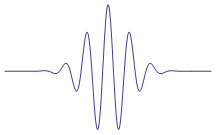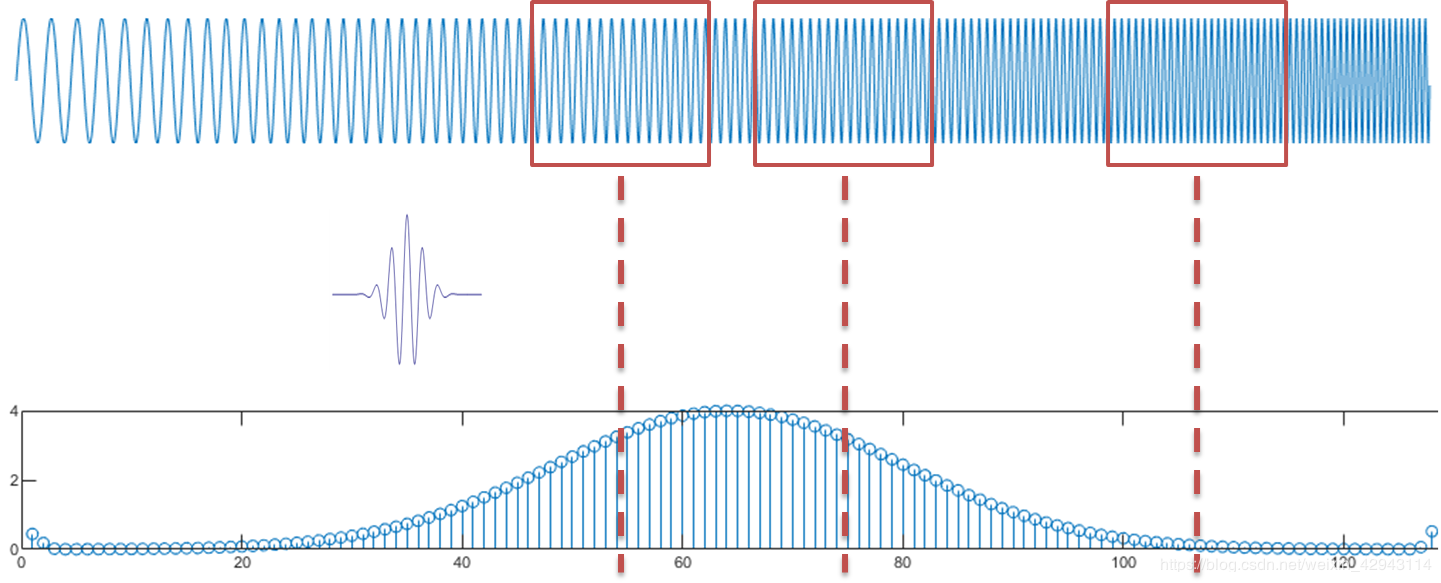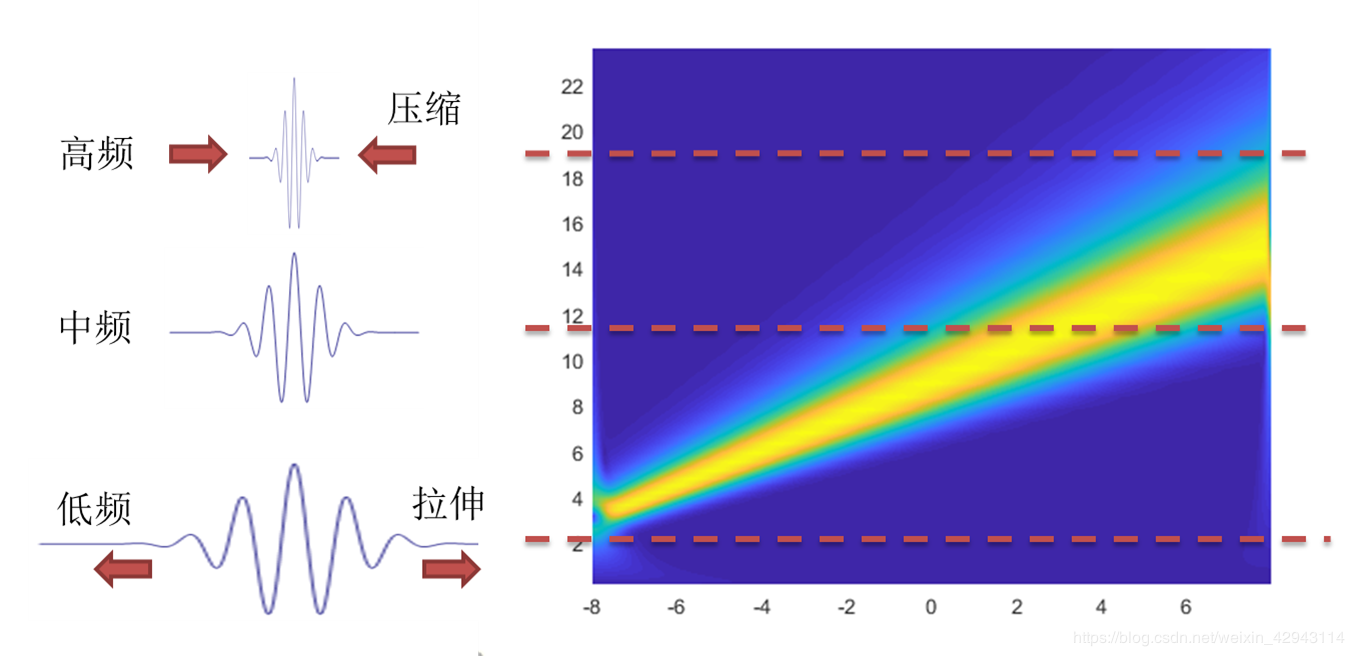scale * f=Fs * wcf


## 3. cwt的Matlab实现

matlab自带的有两种实现方式，一种是2006年版本推出的函数cwt，一种是2016年版本推出的函数cwt。两个函数名称相同，用法不同。

coefs = cwt(x,scales,'wname')


[wt,f] = cwt(x,wname,fs)


https://ww2.mathworks.cn/help/wavelet/gs/choose-a-wavelet.html

% 定义信号信息
fs=2^6;    %采样频率
dt=1/fs;    %时间精度
timestart=-8;
timeend=8;
t=(0:(timeend-timestart)/dt-1)*dt+timestart;
L=length(t);

z=4*sin(2*pi*linspace(6,12,L).*t);

%matlab自带的小波变换
%新版本
figure(1)
[wt,f,coi] = cwt(z,'amor',fs);


% 定义信号信息
fs=2^6;    %采样频率
dt=1/fs;    %时间精度
timestart=-8;
timeend=8;
t=(0:(timeend-timestart)/dt-1)*dt+timestart;
L=length(t);

z=4*sin(2*pi*linspace(6,12,L).*t);
%旧版本
wavename='cmor1-3'; %可变参数，分别为cmor的
%举一个频率转尺度的例子
fmin=2;
fmax=20;
df=0.1;

f=fmin:df:fmax-df;%预期的频率
wcf=centfrq(wavename); %小波的中心频率
scal=fs*wcf./f;%利用频率转换尺度
coefs = cwt(z,scal,wavename);
figure(2)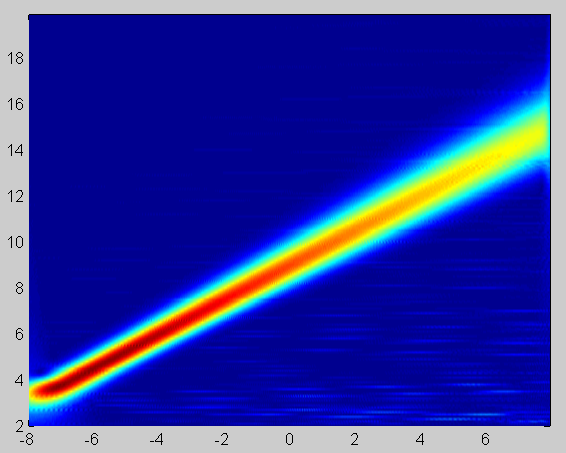cmor小波最终得到的coefs小波系数，是一个复数矩阵，绝对值abs()代表信号的幅值，角度angle()代表信号的相位。一般用小波系数的实部real()来同时表示信号正负变化。

fs=2^6;    %采样频率
dt=1/fs;    %时间精度
timestart=-8;
timeend=8;
t=(0:(timeend-timestart)/dt-1)*dt+timestart;
L=length(t);
z=4*sin(2*pi*linspace(6,12,L).*t);

%定义计算范围和精度
fmin=2;
fmax=20;
df=0.1;
totalscal=(fmax-fmin)/df;
f=fmin:df:fmax-df;%预期的频率

%自己实现的小波函数
coefs2=cwt_cmor(z,1,3,f,fs);
figure(3)

%后面是函数
function coefs=cwt_cmor(z,Fb,Fc,f,fs)
%1 小波的归一信号准备
z=z(:)';%强行变成y向量，避免前面出错
L=length(z);
%2 计算尺度
scal=fs*Fc./f;

%3计算小波
shuaijian=0.001;%取小波衰减长度为1%
tlow2low=sqrt(Fb*log(1/shuaijian));%单边cmor衰减至1%时的时间长度，惨叫cmor的表达式

%3小波的积分函数
iter=10;%小波函数的区间划分精度
xWAV=linspace(-tlow2low,tlow2low,2^iter);
stepWAV = xWAV(2)-xWAV(1);
val_WAV=cumsum(cmorwavf(-tlow2low,tlow2low,2^iter,Fb,Fc))*stepWAV;
%卷积前准备
xWAV = xWAV-xWAV(1);
xMaxWAV = xWAV(end);
coefs     = zeros(length(scal),L);%预初设coefs

%4小波与信号的卷积
for k = 1:length(scal)    %一个scal一行
a_SIG = scal(k); %a是这一行的尺度函数

j = 1+floor((0:a_SIG*xMaxWAV)/(a_SIG*stepWAV));
%j的最大值为是确定的，尺度越大，划分的越密。相当于把一个小波拉伸的越长。
if length(j)==1 , j = [1 1]; end

waveinscal = fliplr(val_WAV(j));%把积分值扩展到j区间，然后左右颠倒。f为当下尺度的积分小波函数

%5 最重要的一步 wkeep1取diff(wconv1(ySIG,f))里长度为lenSIG的中间一段
%conv(ySIG,f)卷积。
coefs(k,:) = -sqrt(a_SIG)*wkeep1(diff(conv2(z,waveinscal, 'full')),L);
%
end
end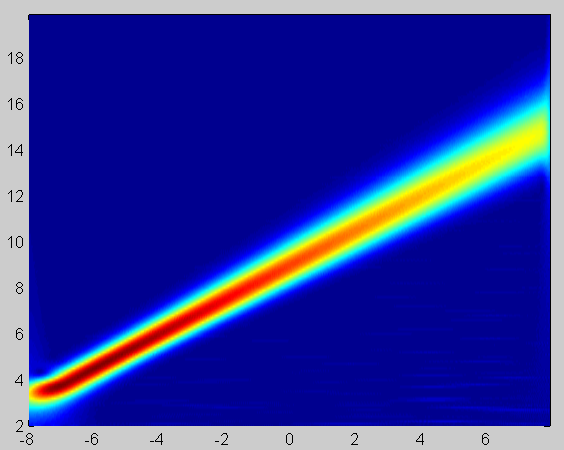#### 带你入门matlab小波分析

03-19

04-281万+

#### matlab时频分析之连续小波变换cwt03-157857

#### MATLAB 利用小波变换进行时频分析

12-211万+

#### 连续小波变换的概念swt,cwt,dwt

02-121155

#### 小波时频图

04-13997

#### 对小波变换中DWT和CWT的理解©️2020 CSDN 皮肤主题: 1024 设计师: 上身试试点击重新获取扫码支付1.余额是钱包充值的虚拟货币，按照1:1的比例进行支付金额的抵扣。
2.余额无法直接购买下载，可以购买VIP、C币套餐、付费专栏及课程。余额充值